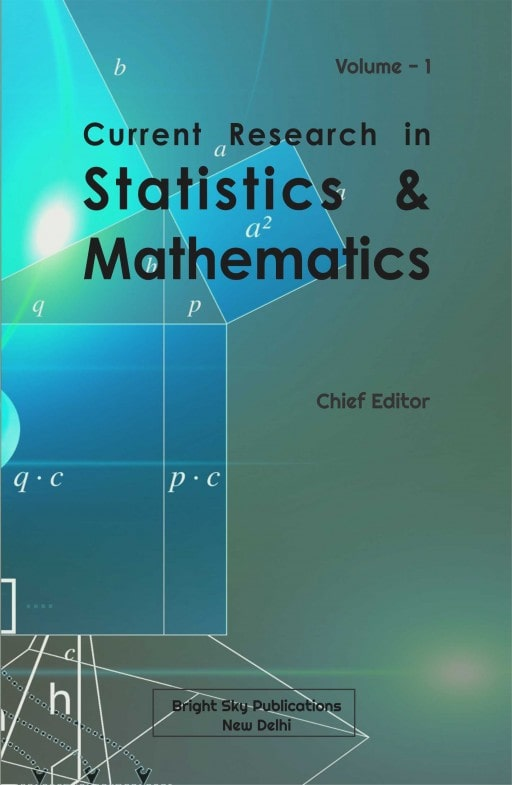# Current Research in Statistics and Mathematics (Volume - 2)

## Mathematics Edited Book | Edited Book on Mathematics

This edited book on mathematics titled "Current Research in Statistics and Mathematics" mainly focuses on various topics such as vedic mathematics, algebra, geometry etc., and the rest are given below in the Scope of the book. This mathematics edited book will be published with ISBN and DOI numbers after following a proper double blind peer reviewed process. All the chapters of this mathematics edited book will be published in a very illustrative manner for easy reader understanding.Book Scope
Vedic Mathematics Algebra Geometry Linear and Multilinear Algebra; Matrix Theory Real Functions Measure and Integration Functions of a Complex Variable Several Complex Variables and Analytic Spaces Ordinary Differential Equations Partial Differential Equations Dynamical Systems and Ergodic Eheory Difference and Functional Equations Fourier Analysis Integral Equations Functional Analysis Operator Theory Calculus of Variations and Optimal Control; Optimization Operations Research Mathematical Programming Differential Geometry General Topology Manifolds and Cell Complexes Global Analysis Analysis on Manifolds Probability Theory and Stochastic Processes Statistics Numerical Analysis Computer Science Mechanics of Particles and Systems Mechanics of Deformable Solids Fluid Mechanics Optics Electromagnetic Theory Quantum Theory and Statistical Mechanics
Chief EditorDr. Manoj Kumar Chande
Professor & Head, Department of Applied Mathematics, SSIPMT, Raipur, Chhattisgarh, India